# CHSE Odisha Class 12 Math Solutions Chapter 11 Differential Equations Ex 11(c)

Odisha State Board Elements of Mathematics Class 12 Solutions CHSE Odisha Chapter 11 Differential Equations Ex 11(c) Textbook Exercise Questions and Answers.

## CHSE Odisha Class 12 Math Solutions Chapter 11 Differential Equations Exercise 11(c)

Find the solutions of the following differential equations:
Question 1.
(x + y) dy + (x – y) dx = 0
Solution:
Given equation can be written as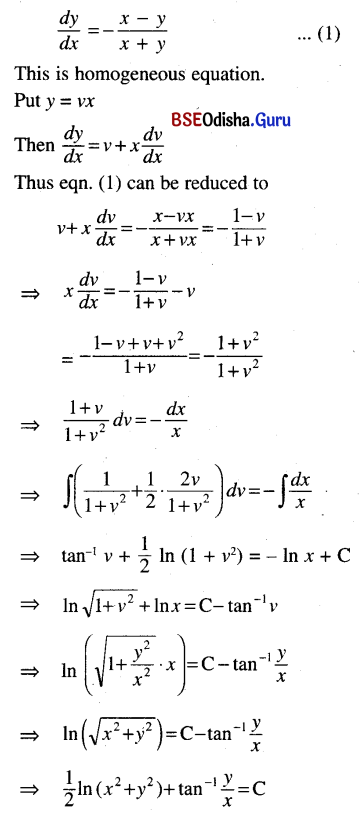Question 2.
$$\frac{d y}{d x}$$ = $$\frac{1}{2}\left(\frac{y}{x}+\frac{y^2}{x^2}\right)$$
Solution: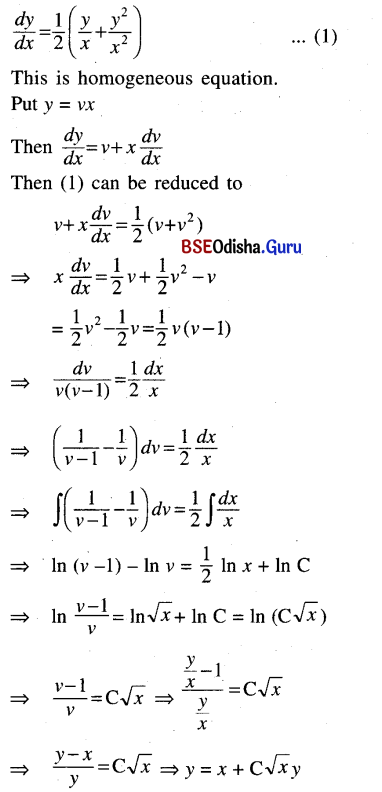Question 3.
(x2 – y2) dx + 2xy dy = 0
Solution:
Given equation can be written as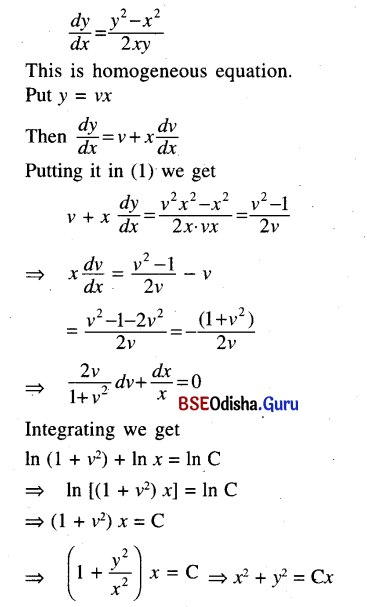Question 4.
x$$\frac{d y}{d x}$$ + $$\sqrt{x^2+y^2}$$ = y
Solution:
Given equation can be written as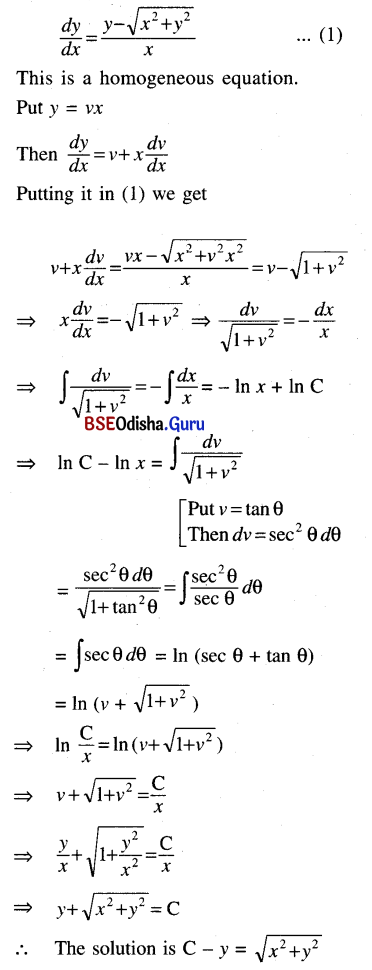Question 5.
x (x + y) dy = (x2 + y2) dx
Solution: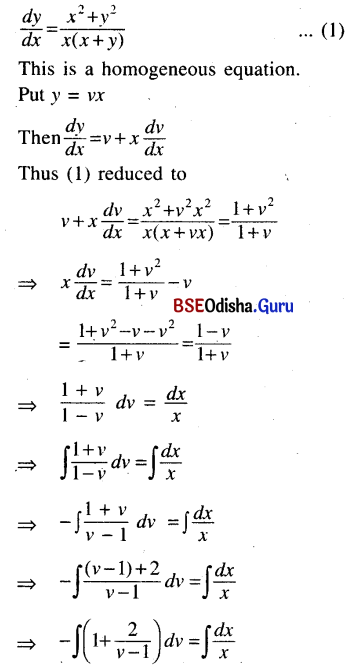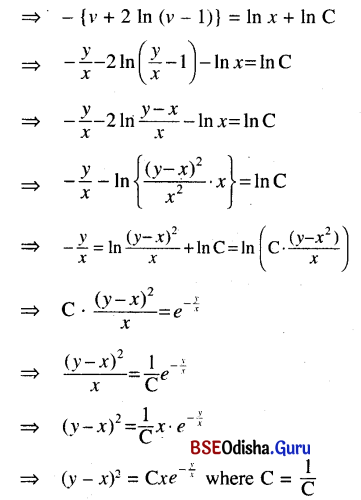This is the required solution.Question 6.
y2 + x2 $$\frac{d y}{d x}$$ = xy $$\frac{d y}{d x}$$
Solution:
Given equation can be written as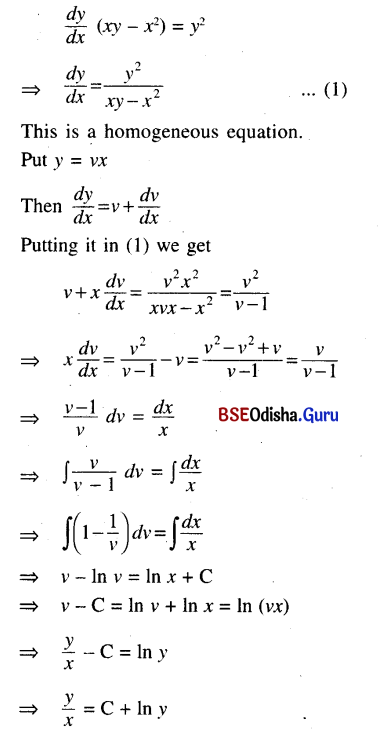This is the required solution.

Question 7.
x sin$$\frac{y}{x}$$ dy = $$\left(y \sin \frac{y}{x}-x\right)$$dx
Solution:
Given equation can be written as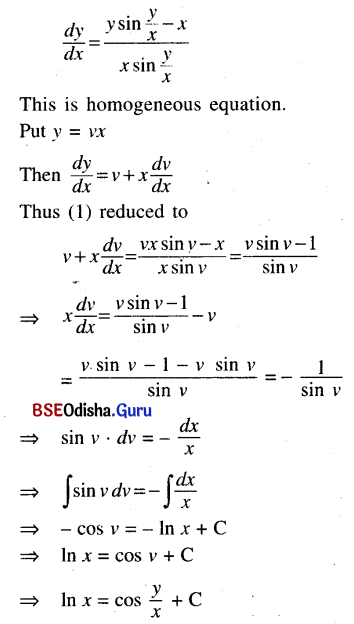Question 8.
x dy – y dx= $$\sqrt{x^2+y^2}$$ dx
Solution: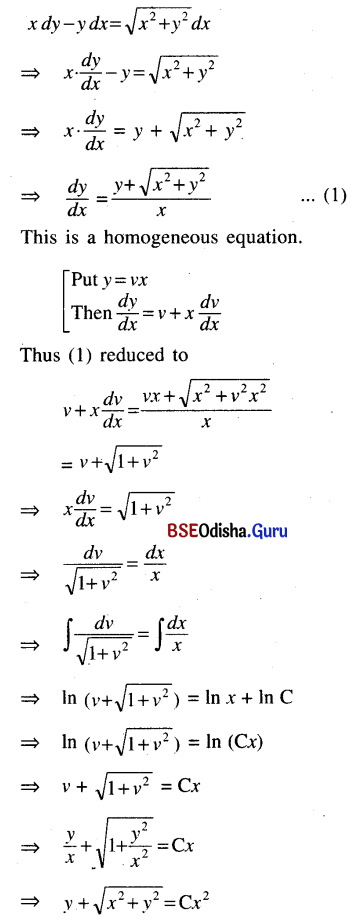This is the required solution.Question 9.
$$\frac{d y}{d x}$$ = $$\frac{y-x+1}{y+x+5}$$
Solution:
Given equation is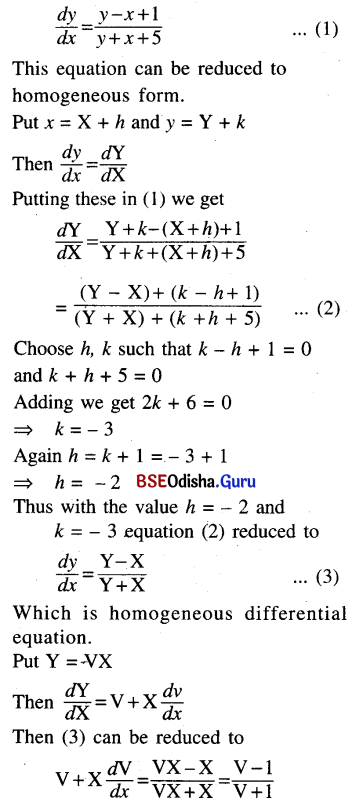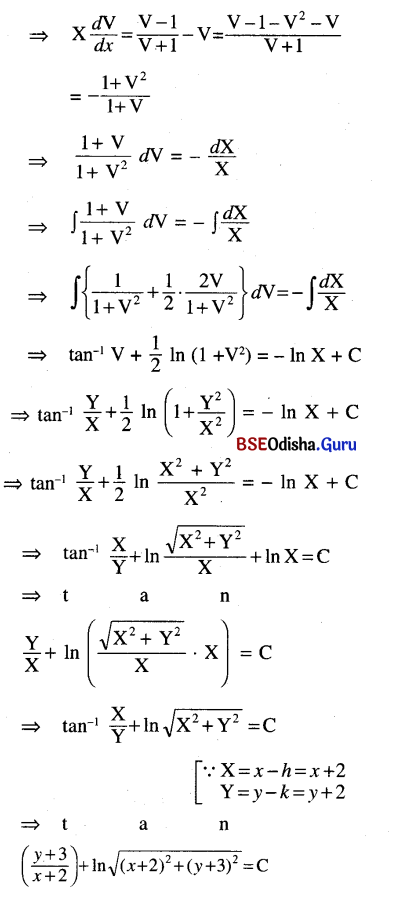This is the required solution.

Question 10.
(x – y) dy = (x + y + 1) dx
Solution:
Given equation can be written as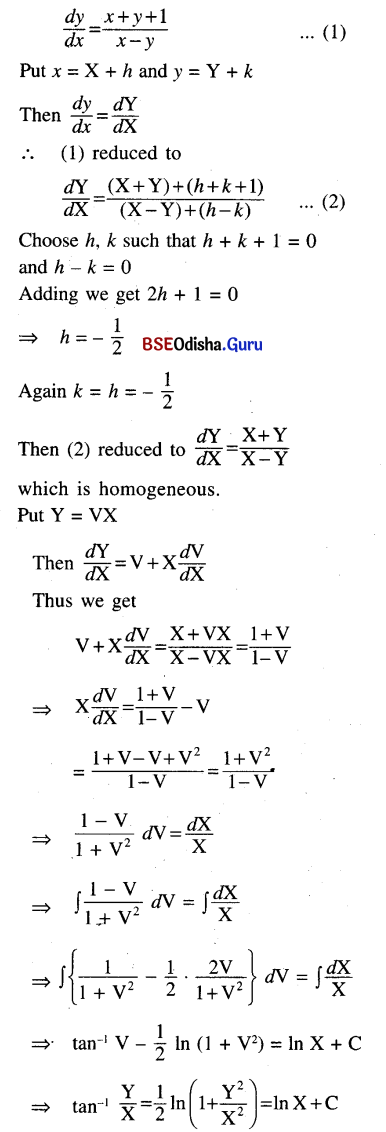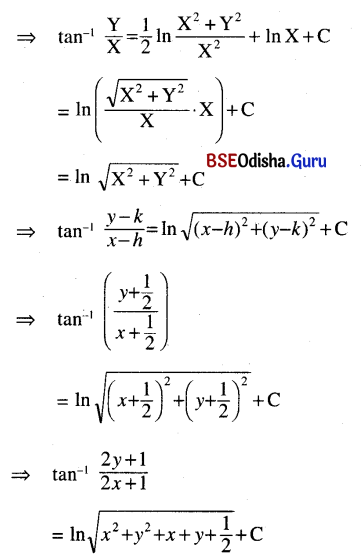This is the required solution.

Question 11.
(x – y – 2) dx + (x – 2y – 3) dy = 0
Solution:
Given equation can be written as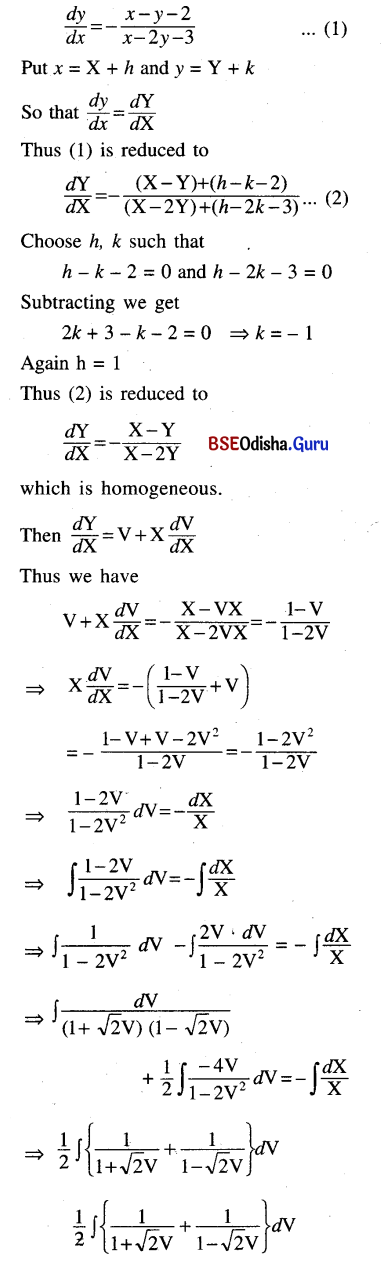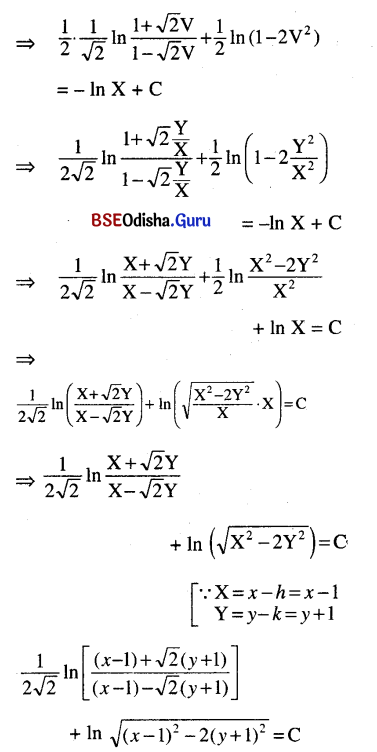This is the required solution.Question 12.
$$\frac{d y}{d x}$$ = $$\frac{3 x-7 y+7}{3 y-7 x-3}$$
Solution:
Given equation can be written as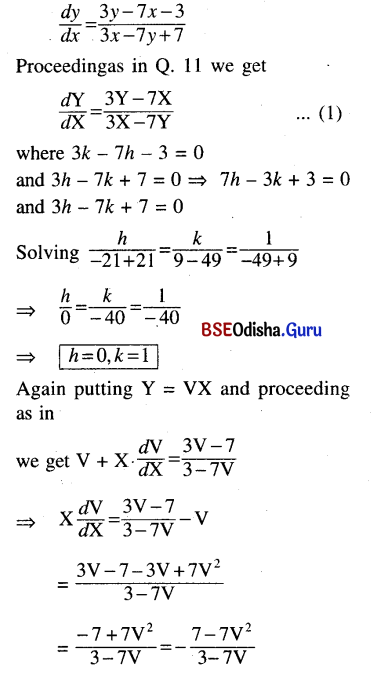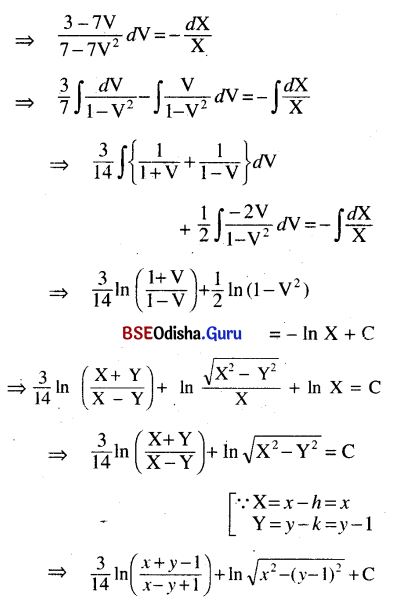This is the required solution.

Question 13.
(2x + y + 1) dx + (4x + 2y – 1) dy = 0
Solution:
Given equation can be written as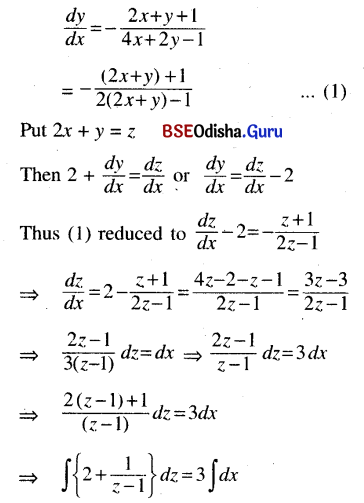⇒ 2z + ln (z – 1) = 3x + C
⇒ 2 (2x + y) + ln (2x + y – 1 ) = 3x + C
⇒ (x + 2y) + ln (2x + y – 1 ) = C
This is the required solution.

Question 14.
(2x + 3y – 5)$$\frac{d y}{d x}$$ + 3x + 2y – 5 = 0
Solution:
Given equation can be written as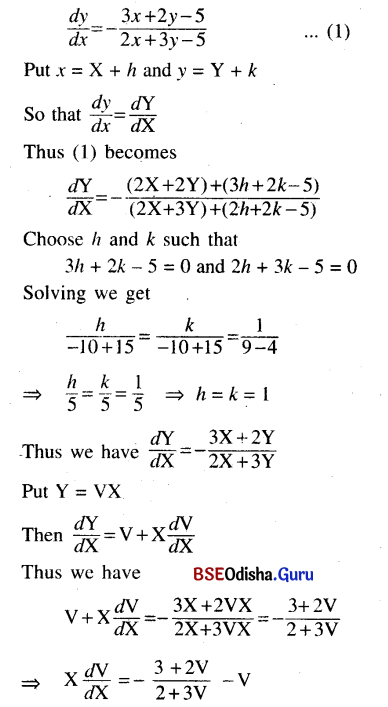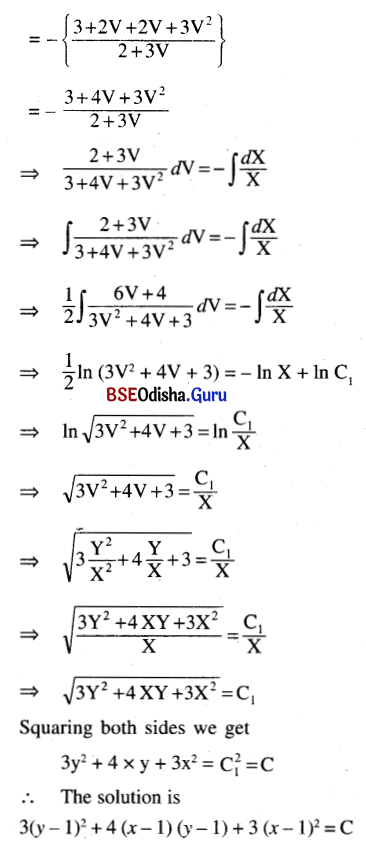Question 15.
(4x + 6y + 5) dx – (2x + 3y + 4) dy = 0
Solution:
Given equation can be written as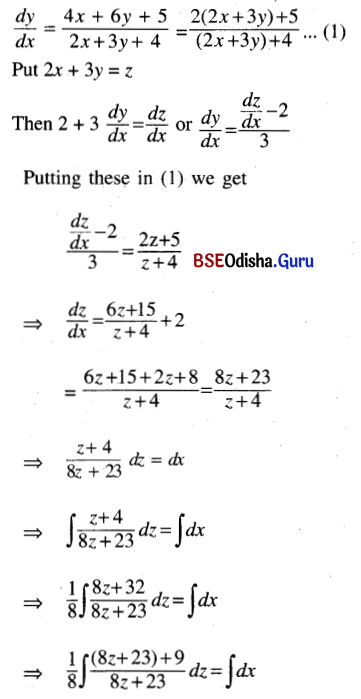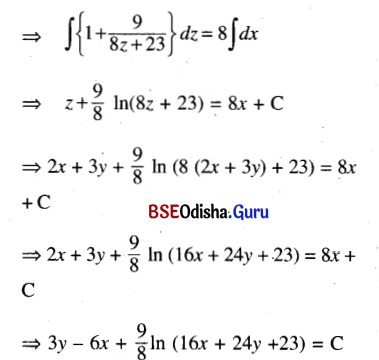This is the required solution.